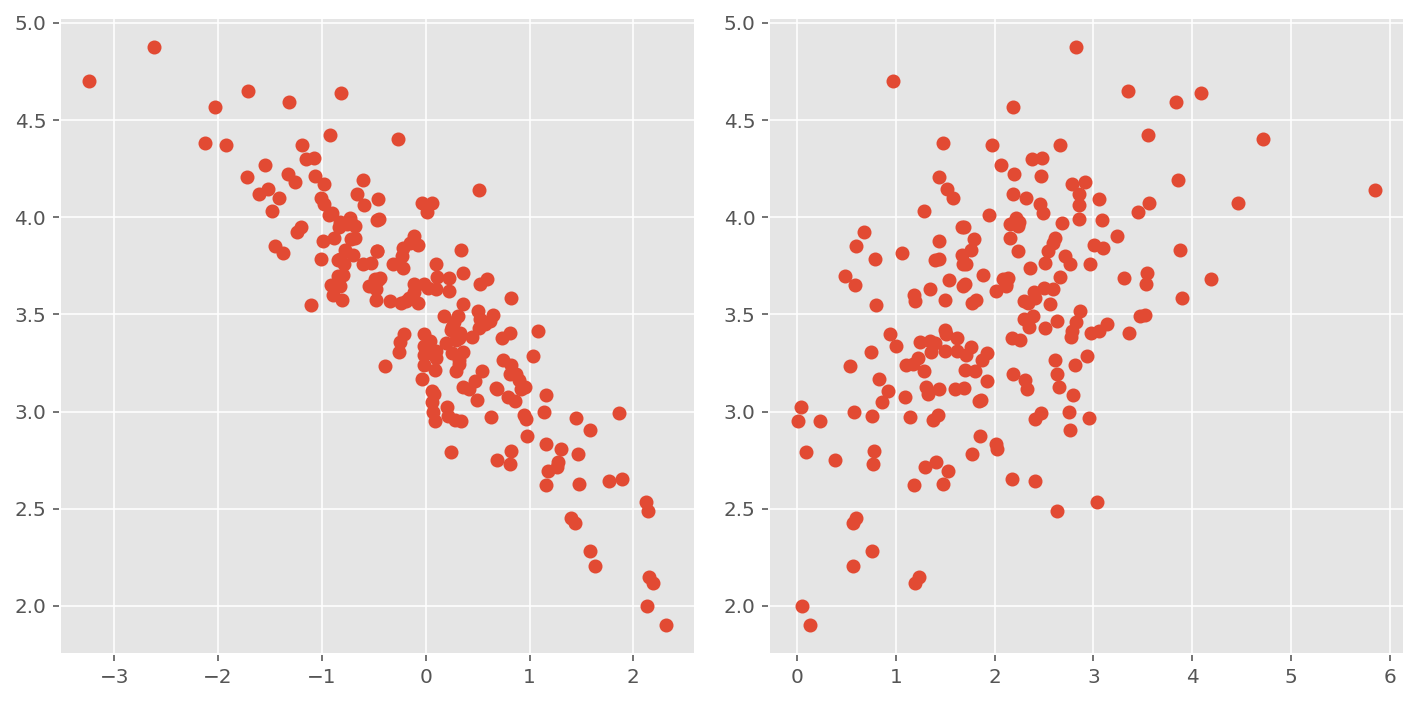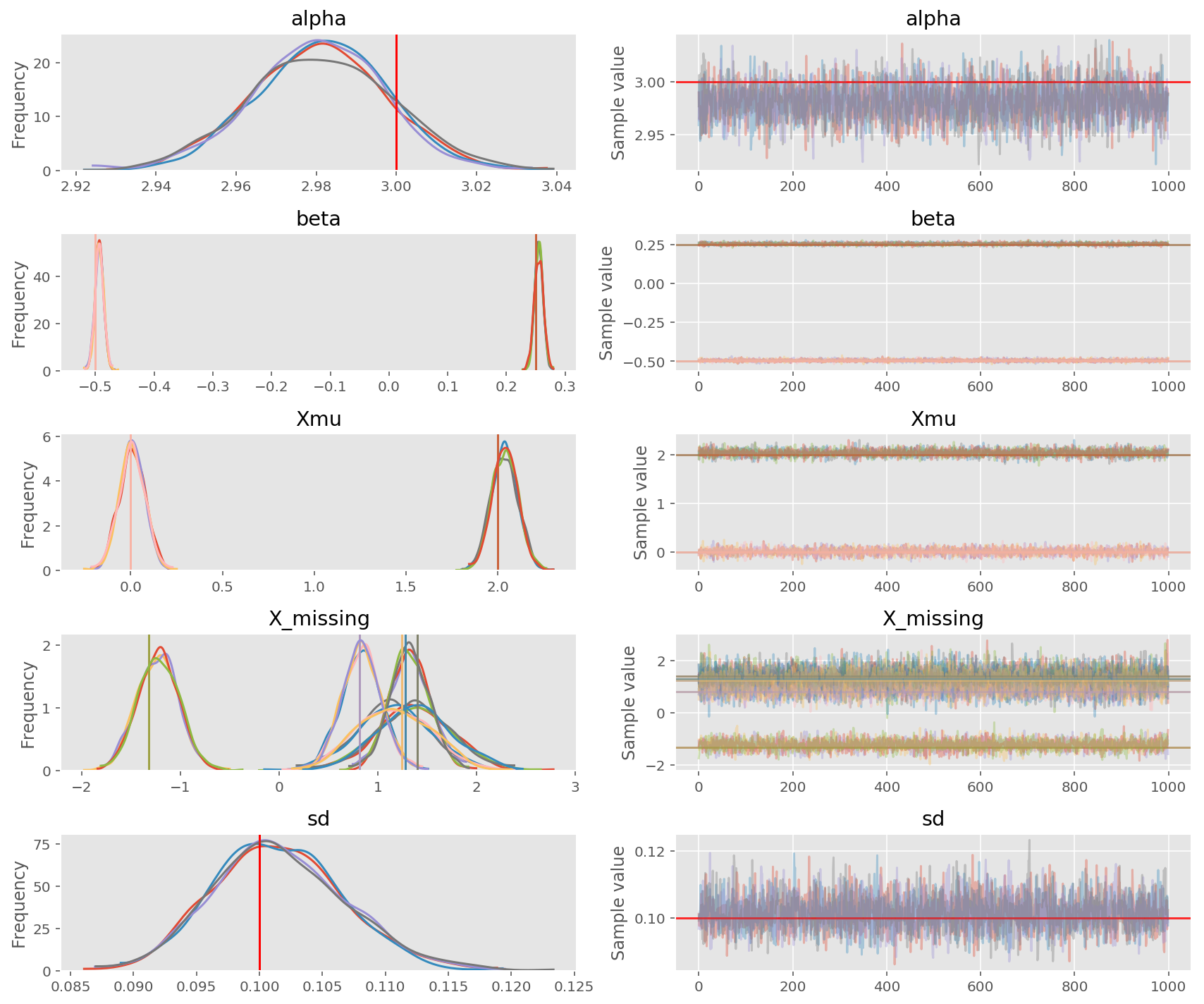2017-10-23

Out-of-sample prediction for linear model with missing data

This blog post is inspired by a user question on Discourse.

The ability to predict new data from old observations has long been considered as one of the golden rules of evaluating science and scientific theory. And in Bayesian modelling, this idea is especially natural: not only it maps new inputs into new outputs the same way as a deterministic model, it does so probabilistically, meaning that you also get the uncertainty of each prediction.

Consider a linear regression problem: the data could be represented as a tuple ($X$, $y$) and we want to find the linear relationship which maps $X\to y$. $X$ is usually referred to as predictors, represented as a matrix (say k * n) so that it is easier to work with using linear algebra. In a setting where we have no missing data, we can write down the linear model as something like this (with weakly informative prior, and the intercept coded in $X$):

\begin{align*} \sigma \sim \textrm{HalfCauchy}(0, 2.5) \\ \beta \sim \textrm{Normal}(0, 10) \\ \textrm{y} \sim \textrm{Normal}(\textrm{X}*\beta, \sigma) \end{align*}

A subtle point here to note here is that values in $X$ are usually considered as given, something trivial to measure, or has little noise (even noiseless). It could be true in some context: for example, $X$ is a dummy-code parameterization of different experimental groups. In general, if $X$ contains continuous measures, it is silly to assume this measurement is noiseless. In psychology, a continous predictor is usually referred to as covariates, which could be analysed with ANCOVA etc. Measurement error in $X$ is difficult to deal with using frequentistic statistics - the uncertainty in covariates just propagate to $y$ (e.g., see propagation of uncertainty in wikipedia). However, in Bayesian Statistics, this is quite natural to model the uncertainty in covariates by considering the observed covariates in $X$ as some noisy realization of some true latent variables/process:

\begin{align*} \sigma \sim \textrm{HalfCauchy}(0, 2.5) \\ \beta \sim \textrm{Normal}(0, 10) \\ \textrm{X_latent} \sim \textrm{Normal}(0, 10) \\ \textrm{X} \sim \textrm{Normal}(\textrm{X_latent}, 1) \\ \textrm{y} \sim \textrm{Normal}(\textrm{X_latent}*\beta, \sigma) \end{align*}

The latter was also shown to be a natural solution for missing data. Here I first generate the data ($X$, $y$), and mask some value in the design matrix $X$ to indicates missing information. $X$ follows a Normal distribution with Xmu=[0, 2], and the missing values are masked using a numpy masked_array.

np.random.seed(42)
n0 = 200
# generate data with missing values
Xmu_ = np.array([0, 2])
x_train = np.random.randn(n0, 2) + Xmu_
beta_ = np.array([-.5, .25])
alpha_ = 3
sd_ = .1
y_train = alpha_ + sd_ * np.random.randn(n0) + np.dot(x_train, beta_.T)

plt.figure(figsize=(10, 5))
gs = gridspec.GridSpec(1, 2)
ax0 = plt.subplot(gs[0, 0])
ax0.plot(x_train[:, 0], y_train, 'o')
ax0 = plt.subplot(gs[0, 1])
ax0.plot(x_train[:, 1], y_train, 'o')
plt.tight_layout();

mask = np.array(np.random.rand(n0, 2) < .015, dtype=int)The above figure shows the linear relationship between the two columns in $X$ and $y$. Now we build this model in PyMC3:

# build model, fit, and check trace
with pm.Model() as model:
alpha = pm.Normal('alpha', mu=0, sd=10)
beta = pm.Normal('beta', mu=0, sd=10, shape=(2,))

Xmu = pm.Normal('Xmu', mu=0, sd=10, shape=(2,))
X_modeled = pm.Normal('X', mu=Xmu, sd=1., observed=X_train)

mu = alpha + tt.dot(X_modeled, beta)
sd = pm.HalfCauchy('sd', beta=10)
y = pm.Normal('y', mu=mu, sd=sd, observed=y_train)

model.free_RVs
[alpha, beta, Xmu, X_missing, sd_log__]

Displaying the free random variables (RVs) in the model, we see that PyMC3 added a new RV X_missing, which we did not declared, into the model. It coded for the missing values in our design matrix $X$.

Now we can sample from the posterior using NUTS and examinate the trace:

# inference
with model:
trace = pm.sample(1000, njobs=4)

pm.traceplot(trace,
lines=dict(alpha=alpha_,
beta=beta_,
sd=sd_,
Xmu=Xmu_,
Auto-assigning NUTS sampler...
53%|█████▎    | 791/1500 [00:19<00:03, 197.17it/s]/Users/jlao/Documents/Github/pymc3/pymc3/step_methods/hmc/nuts.py:451: UserWarning: The acceptance probability in chain 1 does not match the target. It is 0.881618620446, but should be close to 0.8. Try to increase the number of tuning steps.
% (self._chain_id, mean_accept, target_accept))
71%|███████   | 1064/1500 [00:21<00:02, 189.90it/s]/Users/jlao/Documents/Github/pymc3/pymc3/step_methods/hmc/nuts.py:451: UserWarning: The acceptance probability in chain 2 does not match the target. It is 0.894470562819, but should be close to 0.8. Try to increase the number of tuning steps.
% (self._chain_id, mean_accept, target_accept))
95%|█████████▍| 1424/1500 [00:22<00:00, 322.29it/s]/Users/jlao/Documents/Github/pymc3/pymc3/step_methods/hmc/nuts.py:451: UserWarning: The acceptance probability in chain 3 does not match the target. It is 0.882355870377, but should be close to 0.8. Try to increase the number of tuning steps.
% (self._chain_id, mean_accept, target_accept))
100%|██████████| 1500/1500 [00:22<00:00, 65.84it/s]As shown above, the parameters we are usually interested in (e.g., coefficients of the linear model $\beta$) could be recovered from the model nicely. Moreover, it gives an estimation of the missing values in $X$ (also quite close to the real value).

There are some warning of acceptance probability higher than target, but this is nothing to be too alarm of: sampling the missing values usually has a higher acceptance probability.

We can check how good the fit is using posterior prediction checks:

# posterior predictive checks on original data
ppc = pm.sample_ppc(trace, samples=200, model=model)

def plot_predict(ppc_y, y):
plt.figure(figsize=(15, 5))
gs = gridspec.GridSpec(1, 3)
ax0 = plt.subplot(gs[0, 0:2])
ax0.plot(ppc_y.T, color='gray', alpha=.1)
ax0.plot(y, color='r')

ax1 = plt.subplot(gs[0, 2])
for ppc_i in ppc_y:
pm.kdeplot(ppc_i, ax=ax1, color='gray', alpha=.1)
pm.kdeplot(y, ax=ax1, color='r')
plt.tight_layout()
return ax0, ax1

ax0, ax1 = plot_predict(ppc['y'], y_train)